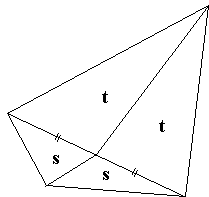# Newton's Theorem: What is it? A Mathematical Droodle

Explanation### Newton's and Léon Anne's Theorems

The applet illustrates the following statement (Newton's theorem]:

The center of the circle inscribed into a quadrilateral lies on the line joining the midpoints of the latter's diagonals.

The line is known as Newton's line. It exists for the quadrilaterals that are not parallelograms.

The statement is an immediate consequence of a theorem of Léon Anne:

### Theorem (Pierre-Léon Anne, 1806-1850)

In any quadrilateral $ABCD$ that is not a parallelogram, the locus of points $O$ such that

$[\Delta AOB] + [ \Delta COD] = [ \Delta BOC] + [ \Delta AOD]$

is Newton's line of the quadrilateral.

Here and in the following $[X]$ denotes the area of shape $X.$

### Proof of Newton's Theorem

For any quadrilateral $ABCD$ circumscribed around a circle $AB + CD = BC + AD$. (This is because two tangents from a point to a circle are equal. To derive the identity, consider four pairs of tangents -- one at each vertex of the quadrilateral.) Multiply that identity by R/2, where R is the radius of the circle, to obtain $[ \Delta AOB] + [ \Delta COD] = [ \Delta BOC] + [ \Delta AOD].$ By the theorem of Léon Anne, the proof is complete.

### Proof 1 of the theorem of Léon Anne[F. G.-M, p. 767]. Since $E$ is the midpoint of the diagonal $BD,$ the lengths of the perpendiculars from $B$ and $D$ onto the line $EF$ are equal. Therefore, say, triangles $BOF$ and $DOF$ have equal areas. Similarly, $[ \Delta AOF] = [ \Delta COF].$ Further,

$[ \Delta AOB] = [ \Delta AFB] + [ \Delta AOF] + [ \Delta BOF]$ and
$[ \Delta DOC] = [ \Delta DFC] - [ \Delta COF] - [ \Delta DOF].$

Therefore

(1)

$[ \Delta AOB] + [ \Delta COD] = [ \Delta AFB] + [ \Delta CFD].$

In the same manner,

$[ \Delta AOD] = [ \Delta AFD] + [ \Delta DOF] - [ \Delta AOF],$ and
$[ \Delta BOC] = [ \Delta BFC] - [ \Delta BOF] + [ \Delta COF].$

Wherefrom

(2)

$[ \Delta AOD] + [ \Delta BOC] = [ \Delta AFD] + [ \Delta CFB].$

But we know that $[ \Delta AFB] = [ \Delta AFD]$ and also $[ \Delta CFD] = [ \Delta CFB],$ which, combined with (1) and (2), yields

(3)

$[ \Delta AOD] + [ \Delta BOC] = [ \Delta AOB] + [ \Delta COD].$

### Proof 2 of the theorem of Léon Anne

[Honsberger, p. 174-175]Since a median bisects the area of a triangle, the midpoints of the diagonals of the quadrilateral lie one the locus in question. An observation due to Basil Rannie makes it clear that the locus is a straight line.

The area of a triangle with a fixed base and a moving apex is the linear function of the Cartesian coordinates of the latter. If the apex is shared by two triangles, their total area is still a linear function of its coordinates. But the level curves of a linear function are straight lines.

### References

1. C. Alsina, R. B. Nelsen, Charming Proofs, MAA, 2010, pp. 116-118
2. F. G.-M., Exercices de Géométrie, Jacques Gabay, 1991
3. R. Honsberger, More Mathematical Morsels, MAA, 1991